Fourier Sine Series

## Linear Methods of Applied Mathematics Evans M. Harrell II and James V. Herod*

version of 26 March 2000

There are three distinct versions of the Fourier series in common use, the "full" Fourier series, the Fourier sine series, and the Fourier cosine series. They are based on slightly different choices of an orthogonal basis set, whether (2.6) for the Fourier sine series, (2.7) for the Fourier cosine series, or (2.8) for the full Fourier series. Notice that while the full set (2.8) contains both sines and cosines, it does not contain as many frequencies as either of the other sets, since the trig functions depend on 2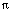n x/L rather than all integer multiples ofx/L. We will also discuss an "exponential" Fourier series, which uses complex functions of the form

exp(2i x/L),

but this is actually the same series as the full Fourier series except that it has been written in a convenient way using exponential functions.

The three different sets do an equally good job of approximating functions in the r.m.s. sense, but their relationship to symmetries is different. For this reason, different sets of Fourier functions will arise in our study of partial differential equations, depending on which kind of boundary conditions are physically appropriate for a given problem.

The full Fourier series consists of functions which are periodic , and are particularly good for representing or approximating periodic functions, that is, functions for which f(x + L) = f(x). Examples include sounds built on a basic frequency with overtones or a physical function depending on an angular variable such as longitude. The Fourier sine series, in contrast, is well adapted to functions which are zero at x=0 and x=L, since all the functions sin(n \pi x/L) have this property. A related but less obvious property that begs for the use of sine functions is an odd symmetry: f(-x) = - f(x). If the function you wish to approximate is an odd function, then the sines are a good candidate for a basis set. Notice that the sines are not only odd under reflection about 0, but also about the other end of the interval, x=L. Fourier cosine series are better suited for even functions. A Fourier sine series represents a function as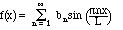(3.a1) for 0 < x < L. In most applications a = 0 < x < L, but this is not strictly necessary. If the lower limit for x is a > 0, then the Fourier sine series can still be used if we change variables from x to x-a, so the basis functions are sin (n(x-a)/L).

The formula for the coefficients bn is the same as in equation 2.14, except that the Fourier sine series uses all integer multiples ofx/L, where L=b-a, not just the even multiples: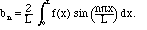(3.a2) Just as for the full Fourier series, the Fourier sine functions are a complete set, and they satisfy some useful identities, which I have collected in a theorem (which is almost identical to the one for the full series). Historically, parts of this theorem were contributed by Fourier, Parseval, Plancherel, Riesz, and Fischer, and in most sources it is considered several theorems. For proofs and further details, refer to Rudin's Real Analysis  or Rogosinski's Fourier Series .

The powerful theorem behind the Fourier sine series III.3a.

If f(x) is square-integrable on an interval [a,b], then

a) All of the coefficients bn of (3.a2) are well defined.

b) All of the coefficients bn are uniquely determined by f(x) and depend linearly on f(x).

c) The series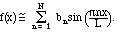converges to f(x) in the r.m.s. sense. In other words,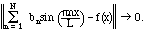We shall express this by writing(Remember, however, that this series converges in a mean sense, and not necessarily at any given point.) It is also true that it converges a.e.

d)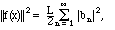and the right side is guaranteed to converge.

e) If g is a second square-integrable function, with Fourier sine coefficients b-tilde, then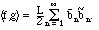This is known as the Parseval formula  for the Fourier sine series.

Conversely, given a square-summable sequence bn, i.e., real or complex numbers such that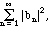is finite, they determine a square integrable function f(x) uniquely a.e. such that all the statements of this theorem hold.

In addition, if f(x) is piecewise continuous, then Theorem III.6 likewise holds, except at the end points of the interval. If 0 < x < L, the Fourier sine series will converge to the average of the right and left limiting values of f at x. When x=0 or L, however, the Fourier sine series will always converge to 0, since all the sine functions are 0 at those two points.

Compare with the Fourier cosine series

Compare with the full Fourier series

Compare with the exponential Fourier series